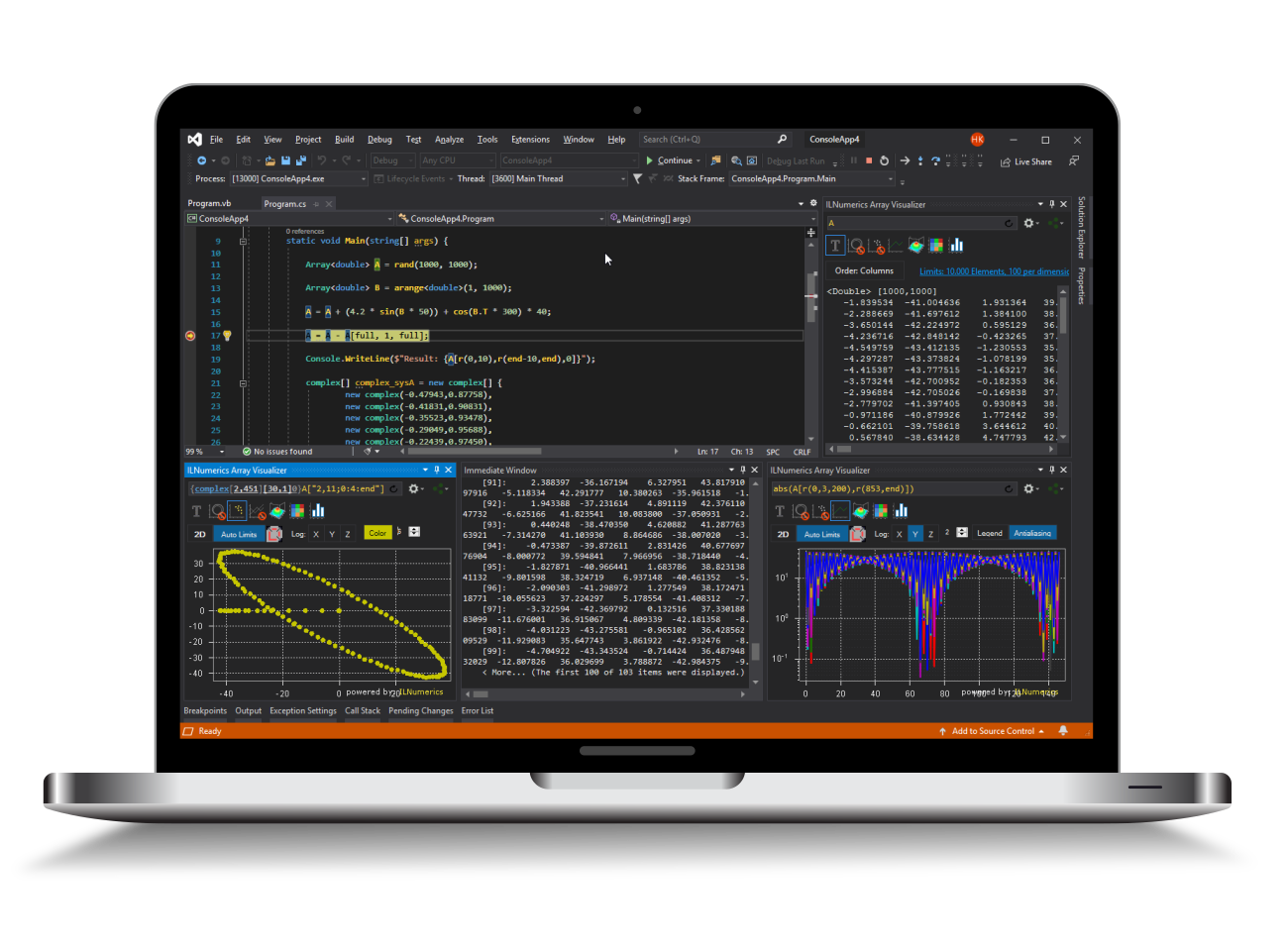Industrial Data Science
in C# and .NET:
Simple. Fast. Reliable.ILNumerics - Technical Computing

Modern High Performance Tools for Technical

Computing and Visualization in Industry and Science

tgt

# Special Functions in .NET (C#, Visual Basic)

ILNumerics offers a range of special functions to extend the computing capability out of the box. Click on the name of a function in order to go to the corresponding API documentation.

Method name Short description
Special Functions
gamma Evaluates the Gamma function.
gammaLog Evaluates the natural logarithm of the Gamma function.
factorial Calculates the Factorial.

factorialLog

Calculates the natural logarithm of Factorial.
beta Evaluates the componentwise Beta function.
binomialCoefficients Calculates the Binomial coefficients.
binomialCoefficientsLog Calculates the natural logarithm of Binomial coefficients.
gammaIncomplete Evaluates the lower incomplete Gamma function.
betaIncomplete Evaluates the incomplete Beta function.
errorFunction Evaluates the (Gauss) error function.
errorFunctionComplement Evaluates of the complementary (Gauss) error function.
errorFunctionInverse Evaluates  the inverse of the (Gauss) error function.
logistic Evaluates the sigmoid logistic function.
logit Evaluates the inverse of the sigmoid logistic function.
besselJ0 Evaluates the Bessel function of the first kind, integer order 0 of the argument.
besselJ1 Evaluates the Bessel function of the first kind, integer order 1 of the argument.
besselJn Evaluates the Bessel function of the first kind, integer order n-th of the argument.
besselY0 Evaluates the Bessel function of the second kind, integer order 0 of the argument.
besselY1 Evaluates the Bessel function of the second kind, integer order 1 of the argument.
besselYn Evaluates the Bessel function of the second kind, integer order n-th of the argument.

besselModifiedI0

Evaluates the modified Bessel function of the first kind, integer order 0 of the argument.

besselModifiedI1 Evaluates the modified Bessel function of the first kind, integer order 1 of the argument.
besselModifiedIn Evaluates the modified Bessel function of the first kind, integer order n-th of the argument.
besselModifiedK0 Evaluates the modified Bessel function of the second kind, integer order 0 of the argument.
besselModifiedK1 Evaluates the modified Bessel function of the second kind, integer order 1 of the argument.
besselModifiedKn Evaluates the modified Bessel function of the second kind, integer order n-th of the argument.
diGamma Computes the Digamma function.